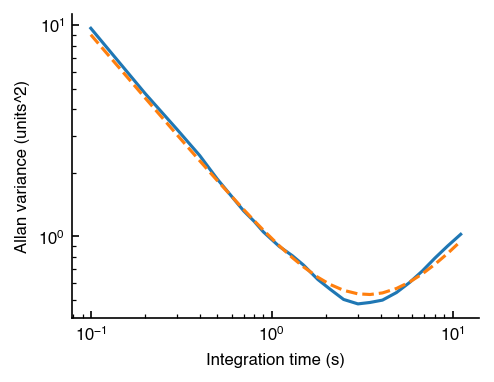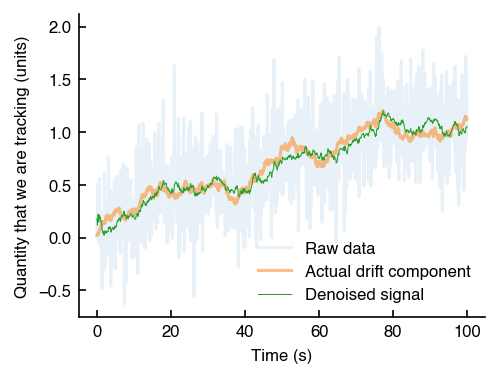# How to denoise a 1-D signal with a Kalman Filter with Python

I frequently need to denoise a signal that is the sum of a noise and drift process. I'm going to provide a quick little Python tutorial (with some code you can copy-paste) that you can use to denoise noise and drift in your experiments.

Let's suppose we have some measurement $$x[t]$$ in some arbitary units (e.g., volts) that is measured once every $$dt$$. Our measurement is the sum of a noise and drift process:

$$x[t] = x_N[t] + x_K[t]$$

We define the noise process

$$x_{N}[t] = w_N \sqrt{dt}$$

where $$w_N$$ is a Gaussian random variable with variance $$N^2$$ and dimensionality [units/√Hz]. We also have a drift process, which is a random walk

$$x_{K}[t] = x_{K}[t-dt] + w_K / \sqrt{dt}$$

where $$w_K$$ is a Gaussian random variable with variance $$K^2$$ and dimensionality [units/√s]

In general, we usually do not know the values of N and K a priori, so we need to extract them. I like to use the Allan variance. The Allan variance can be calculated in Python with the following snippet

import numpy as np

def oavar(data, rate, numpoints=30):
"""Return the Allan variance of the data

data: The data with dimensionality [units]
rate: The sampling rate of the data [e.g., 10 Hz]
(this is 1/dt)

returns:
tau: An array of integration times
oavar: An array of the Allan variance evaluated at each tau
"""

x = np.cumsum(data) / rate
ms = generate_integration_multiples(len(x), numpoints=numpoints)
oavars = np.empty(len(ms))
for i, m in enumerate(ms):
oavars[i] = (
(x[2*m:] - 2*x[m:-m] + x[:-2*m])**2
).mean() / (2*m**2)

return ms / rate, oavars

def generate_integration_multiples(length, numpoints, max_ratio=1/9):
"""Return logarithmically-spaced integers between 1 and the
largest intagration time"""
ms = np.unique(np.logspace(
0, np.log10(length * max_ratio), numpoints
).astype(int))
return ms


The theoretical Allan variance of our noise process is

$$\sigma_N^2(\tau) = N^2 / \tau$$

and the theoretical Allan variance of our drift process is

$$\sigma_K^2(\tau) = K^2 \tau/3$$

So the overall theoretical Allan variance of our measurement will be

$$\sigma^2(\tau) = N^2 / \tau + K^2 \tau/3$$

and we can extract the values of N and K with a least-squares fit. Note that the estimate will be the best if we do our least-squares in a logarithmic scaling of $$\tau$$ andn $$\sigma^2$$

from scipy.optimize import curve_fit

# Only need this if you want to plot the Allan deviation fit
# to the Allan deviation of the data
import matplotlib.pyplot as plt

def NKfit(tau, N, K):
"""Theoretical Allan deviation, linear scaling"""
return N**2 / tau + K**2 * (tau/3)

def ln_NKfit(ln_tau, ln_N, ln_K):
"""Theoretical Allan deviation, logarithmic scaling"""
tau = np.exp(ln_tau)
N, K = np.exp([ln_N, ln_K])

def get_NK(data, rate, show_graph=False):
"""Given a dataset and a sampling rate
taus, oavars = oavar(data, rate)"""

ln_params, ln_varmatrix = (
curve_fit(ln_NKfit, np.log(taus), np.log(oavars))
)

if show_graph:
plt.loglog(taus, oavars)
plt.loglog(taus, NKfit(taus, *np.exp(ln_params)), ls='dashed')

return np.exp(ln_params)


Testing this out with some dummy data:

np.random.seed(42)

N = 1 # units/√Hz
K = .5 # units/√s
rate = 10 # Hz
duration = 100 # s

n_samples = rate * duration

noise_component = np.random.randn(n_samples) / (N * rate**.5)
drift_component = np.random.randn(n_samples).cumsum() * K / rate**1.5
data = noise_component + drift_component

# Now extract N and K
N_fit, K_fit = get_NK(data, rate, show_graph=True)
print(N_fit, K_fit)
#0.9482454912291044 0.48418371069967137The solid blue line is the Allan variance of the data, and the dashed orange line is the fit. As you can see from the output of the code, the value of the noise is about 5% off of the actual value, and the value of teh drift is also about 3% off of the actual value. Not bad!

## Denoising with a Kalman Filter

Finally, let's denoise with a Kalman Filter

# Pre-allocate space for output
output = np.empty(len(data))

# Calculate Kalman filter parameters
process_noise = K**2 * dt
measurement_noise = N**2 / dt

# Initialize state and uncertainty
state = data
covariance = measurement_noise

dt = 1/rate

for index, measurement in enumerate(data):
# Increment our uncertainty of our value
covariance += process_noise

# Calculate Kalman gain (how much we should trust our next sensor measurement relative to previous estimate)
kalman_gain = covariance / (covariance + measurement_noise)

# Using Kalman gain, update our estimate with the next measurement and decrease our covariance
state += kalman_gain * (measurement - state)
covariance = (1 - kalman_gain) * covariance

output[index] = state


Let's take a look at what this looks like for our sample dataNot bad! Note that if there were no noise (i.e., $$N = 0$$), then the orange and green curves would lie on top of each other. The more noise we have, the more the two curves will be different from each other.

Note also that in this case we assumed that the signal we are trying to reconstruct is a simple random walk. If there was any other structure to the signal (e.g., some momentum, etc...), we could do a better job at denoising, but that would require a 2-dimensional Kalman Filter. For that, you should probably use a Python Kalman filter library.

## Copy-Paste Code

Ok. I promsed some copy-paste code. Here it is.

import numpy as np from scipy.optimize import curve_fit

import numpy as np
from scipy.optimize import curve_fit

def denoise(data):

def oavar(data, rate, numpoints=30):

x = np.cumsum(data)

max_ratio = 1/9
num_points = 30
ms = np.unique(
np.logspace(0, np.log10(len(x) * max_ratio), numpoints
).astype(int))

oavars = np.empty(len(ms))
for i, m in enumerate(ms):
oavars[i] = (
(x[2*m:] - 2*x[m:-m] + x[:-2*m])**2
).mean() / (2*m**2)

return ms / rate, oavars

def ln_NKfit(ln_tau, ln_N, ln_K):
tau = np.exp(ln_tau)
N, K = np.exp([ln_N, ln_K])
oadev = N**2 / tau + K**2 * (tau/3)

def get_NK(data, rate):
taus, oavars = oavar(data, rate)

ln_params, ln_varmatrix = (
curve_fit(ln_NKfit, np.log(taus), np.log(oavars))
)
return np.exp(ln_params)

# Initialize state and uncertainty
state = data
output = np.empty(len(data))

rate = 1 # We can set this to 1, if we're calculating N, K internally
# N and K will just be scaled relative to the sampling rate internally
dt = 1/rate

N, K = get_NK(data, rate)

process_noise = K**2 * dt
measurement_noise = N**2 / dt

covariance = measurement_noise

for index, measurement in enumerate(data):
# 1. Predict state using system's model

covariance += process_noise

# Update
kalman_gain = covariance / (covariance + measurement_noise)

state += kalman_gain * (measurement - state)
covariance = (1 - kalman_gain) * covariance

output[index] = state

return output


and call like

denoised_output = denoise(data)Christ-follower, brother, husband, father, grad student.